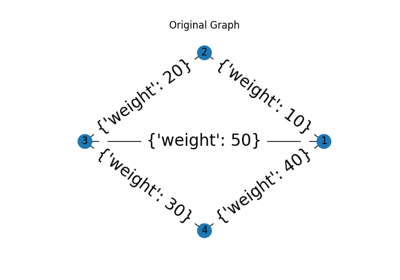# Module: future.graph¶

 skimage.future.graph.cut_normalized(labels, rag) Perform Normalized Graph cut on the Region Adjacency Graph. skimage.future.graph.cut_threshold(labels, …) Combine regions separated by weight less than threshold. Perform hierarchical merging of a RAG. skimage.future.graph.ncut(labels, rag[, …]) Perform Normalized Graph cut on the Region Adjacency Graph. skimage.future.graph.rag_boundary(labels, …) Comouter RAG based on region boundaries Compute the Region Adjacency Graph using mean colors. skimage.future.graph.show_rag(labels, rag, image) Show a Region Adjacency Graph on an image. skimage.future.graph.RAG([label_image, …]) The Region Adjacency Graph (RAG) of an image, subclasses networx.Graph

## cut_normalized¶

skimage.future.graph.cut_normalized(labels, rag, thresh=0.001, num_cuts=10, in_place=True, max_edge=1.0, *, random_state=None)[source]

Perform Normalized Graph cut on the Region Adjacency Graph.

Given an image’s labels and its similarity RAG, recursively perform a 2-way normalized cut on it. All nodes belonging to a subgraph that cannot be cut further are assigned a unique label in the output.

Parameters
labelsndarray

The array of labels.

ragRAG

threshfloat

The threshold. A subgraph won’t be further subdivided if the value of the N-cut exceeds thresh.

num_cutsint

The number or N-cuts to perform before determining the optimal one.

in_placebool

If set, modifies rag in place. For each node n the function will set a new attribute rag.nodes[n]['ncut label'].

max_edgefloat, optional

The maximum possible value of an edge in the RAG. This corresponds to an edge between identical regions. This is used to put self edges in the RAG.

random_state{None, int, numpy.random.Generator}, optional

If random_state is None the numpy.random.Generator singleton is used. If random_state is an int, a new Generator instance is used, seeded with random_state. If random_state is already a Generator instance then that instance is used.

The random_state is used for the starting point of scipy.sparse.linalg.eigsh.

Returns
outndarray

The new labeled array.

References

1

Shi, J.; Malik, J., “Normalized cuts and image segmentation”, Pattern Analysis and Machine Intelligence, IEEE Transactions on, vol. 22, no. 8, pp. 888-905, August 2000.

Examples

>>> from skimage import data, segmentation
>>> from skimage.future import graph
>>> img = data.astronaut()
>>> labels = segmentation.slic(img)
>>> rag = graph.rag_mean_color(img, labels, mode='similarity')
>>> new_labels = graph.cut_normalized(labels, rag)


### Examples using skimage.future.graph.cut_normalized¶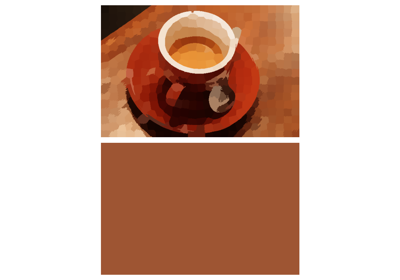## cut_threshold¶

skimage.future.graph.cut_threshold(labels, rag, thresh, in_place=True)[source]

Combine regions separated by weight less than threshold.

Given an image’s labels and its RAG, output new labels by combining regions whose nodes are separated by a weight less than the given threshold.

Parameters
labelsndarray

The array of labels.

ragRAG

threshfloat

The threshold. Regions connected by edges with smaller weights are combined.

in_placebool

If set, modifies rag in place. The function will remove the edges with weights less that thresh. If set to False the function makes a copy of rag before proceeding.

Returns
outndarray

The new labelled array.

References

1

Alain Tremeau and Philippe Colantoni “Regions Adjacency Graph Applied To Color Image Segmentation” DOI:10.1109/83.841950

Examples

>>> from skimage import data, segmentation
>>> from skimage.future import graph
>>> img = data.astronaut()
>>> labels = segmentation.slic(img)
>>> rag = graph.rag_mean_color(img, labels)
>>> new_labels = graph.cut_threshold(labels, rag, 10)


### Examples using skimage.future.graph.cut_threshold¶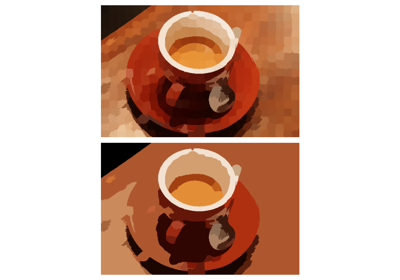## merge_hierarchical¶

skimage.future.graph.merge_hierarchical(labels, rag, thresh, rag_copy, in_place_merge, merge_func, weight_func)[source]

Perform hierarchical merging of a RAG.

Greedily merges the most similar pair of nodes until no edges lower than thresh remain.

Parameters
labelsndarray

The array of labels.

ragRAG

threshfloat

Regions connected by an edge with weight smaller than thresh are merged.

rag_copybool

If set, the RAG copied before modifying.

in_place_mergebool

If set, the nodes are merged in place. Otherwise, a new node is created for each merge..

merge_funccallable

This function is called before merging two nodes. For the RAG graph while merging src and dst, it is called as follows merge_func(graph, src, dst).

weight_funccallable

The function to compute the new weights of the nodes adjacent to the merged node. This is directly supplied as the argument weight_func to merge_nodes.

Returns
outndarray

The new labeled array.

### Examples using skimage.future.graph.merge_hierarchical¶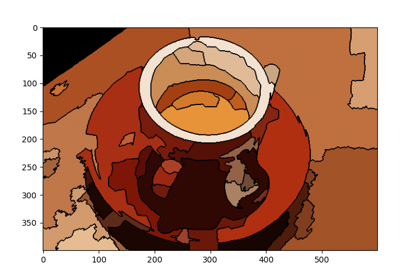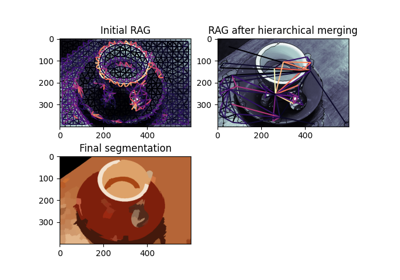## ncut¶

skimage.future.graph.ncut(labels, rag, thresh=0.001, num_cuts=10, in_place=True, max_edge=1.0, *, random_state=None)[source]

Perform Normalized Graph cut on the Region Adjacency Graph.

Given an image’s labels and its similarity RAG, recursively perform a 2-way normalized cut on it. All nodes belonging to a subgraph that cannot be cut further are assigned a unique label in the output.

Parameters
labelsndarray

The array of labels.

ragRAG

threshfloat

The threshold. A subgraph won’t be further subdivided if the value of the N-cut exceeds thresh.

num_cutsint

The number or N-cuts to perform before determining the optimal one.

in_placebool

If set, modifies rag in place. For each node n the function will set a new attribute rag.nodes[n]['ncut label'].

max_edgefloat, optional

The maximum possible value of an edge in the RAG. This corresponds to an edge between identical regions. This is used to put self edges in the RAG.

random_state{None, int, numpy.random.Generator}, optional

If random_state is None the numpy.random.Generator singleton is used. If random_state is an int, a new Generator instance is used, seeded with random_state. If random_state is already a Generator instance then that instance is used.

The random_state is used for the starting point of scipy.sparse.linalg.eigsh.

Returns
outndarray

The new labeled array.

References

1

Shi, J.; Malik, J., “Normalized cuts and image segmentation”, Pattern Analysis and Machine Intelligence, IEEE Transactions on, vol. 22, no. 8, pp. 888-905, August 2000.

Examples

>>> from skimage import data, segmentation
>>> from skimage.future import graph
>>> img = data.astronaut()
>>> labels = segmentation.slic(img)
>>> rag = graph.rag_mean_color(img, labels, mode='similarity')
>>> new_labels = graph.cut_normalized(labels, rag)


## rag_boundary¶

skimage.future.graph.rag_boundary(labels, edge_map, connectivity=2)[source]

Comouter RAG based on region boundaries

Given an image’s initial segmentation and its edge map this method constructs the corresponding Region Adjacency Graph (RAG). Each node in the RAG represents a set of pixels within the image with the same label in labels. The weight between two adjacent regions is the average value in edge_map along their boundary.

labelsndarray

The labelled image.

edge_mapndarray

This should have the same shape as that of labels. For all pixels along the boundary between 2 adjacent regions, the average value of the corresponding pixels in edge_map is the edge weight between them.

connectivityint, optional

Pixels with a squared distance less than connectivity from each other are considered adjacent. It can range from 1 to labels.ndim. Its behavior is the same as connectivity parameter in scipy.ndimage.generate_binary_structure.

Examples

>>> from skimage import data, segmentation, filters, color
>>> from skimage.future import graph
>>> img = data.chelsea()
>>> labels = segmentation.slic(img)
>>> edge_map = filters.sobel(color.rgb2gray(img))
>>> rag = graph.rag_boundary(labels, edge_map)


### Examples using skimage.future.graph.rag_boundary¶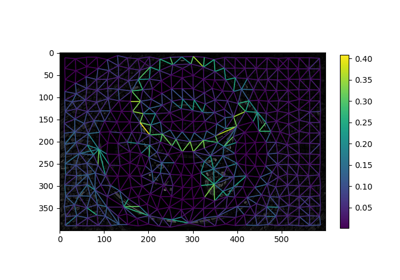## rag_mean_color¶

skimage.future.graph.rag_mean_color(image, labels, connectivity=2, mode='distance', sigma=255.0)[source]

Compute the Region Adjacency Graph using mean colors.

Given an image and its initial segmentation, this method constructs the corresponding Region Adjacency Graph (RAG). Each node in the RAG represents a set of pixels within image with the same label in labels. The weight between two adjacent regions represents how similar or dissimilar two regions are depending on the mode parameter.

Parameters
imagendarray, shape(M, N, […, P,] 3)

Input image.

labelsndarray, shape(M, N, […, P])

The labelled image. This should have one dimension less than image. If image has dimensions (M, N, 3) labels should have dimensions (M, N).

connectivityint, optional

Pixels with a squared distance less than connectivity from each other are considered adjacent. It can range from 1 to labels.ndim. Its behavior is the same as connectivity parameter in scipy.ndimage.generate_binary_structure.

mode{‘distance’, ‘similarity’}, optional

The strategy to assign edge weights.

‘distance’ : The weight between two adjacent regions is the $$|c_1 - c_2|$$, where $$c_1$$ and $$c_2$$ are the mean colors of the two regions. It represents the Euclidean distance in their average color.

‘similarity’ : The weight between two adjacent is $$e^{-d^2/sigma}$$ where $$d=|c_1 - c_2|$$, where $$c_1$$ and $$c_2$$ are the mean colors of the two regions. It represents how similar two regions are.

sigmafloat, optional

Used for computation when mode is “similarity”. It governs how close to each other two colors should be, for their corresponding edge weight to be significant. A very large value of sigma could make any two colors behave as though they were similar.

Returns
outRAG

References

1

Alain Tremeau and Philippe Colantoni “Regions Adjacency Graph Applied To Color Image Segmentation” DOI:10.1109/83.841950

Examples

>>> from skimage import data, segmentation
>>> from skimage.future import graph
>>> img = data.astronaut()
>>> labels = segmentation.slic(img)
>>> rag = graph.rag_mean_color(img, labels)


### Examples using skimage.future.graph.rag_mean_color¶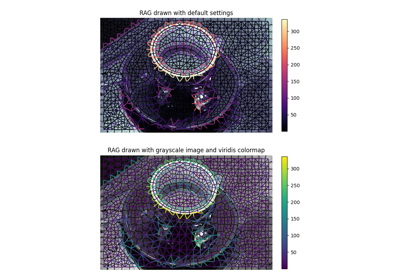## show_rag¶

skimage.future.graph.show_rag(labels, rag, image, border_color='black', edge_width=1.5, edge_cmap='magma', img_cmap='bone', in_place=True, ax=None)[source]

Show a Region Adjacency Graph on an image.

Given a labelled image and its corresponding RAG, show the nodes and edges of the RAG on the image with the specified colors. Edges are displayed between the centroid of the 2 adjacent regions in the image.

Parameters
labelsndarray, shape (M, N)

The labelled image.

ragRAG

imagendarray, shape (M, N[, 3])

Input image. If colormap is None, the image should be in RGB format.

border_colorcolor spec, optional

Color with which the borders between regions are drawn.

edge_widthfloat, optional

The thickness with which the RAG edges are drawn.

edge_cmapmatplotlib.colors.Colormap, optional

Any matplotlib colormap with which the edges are drawn.

img_cmapmatplotlib.colors.Colormap, optional

Any matplotlib colormap with which the image is draw. If set to None the image is drawn as it is.

in_placebool, optional

If set, the RAG is modified in place. For each node n the function will set a new attribute rag.nodes[n]['centroid'].

axmatplotlib.axes.Axes, optional

The axes to draw on. If not specified, new axes are created and drawn on.

Returns
lcmatplotlib.collections.LineCollection

A colection of lines that represent the edges of the graph. It can be passed to the matplotlib.figure.Figure.colorbar() function.

Examples

>>> from skimage import data, segmentation
>>> from skimage.future import graph
>>> import matplotlib.pyplot as plt
>>>
>>> img = data.coffee()
>>> labels = segmentation.slic(img)
>>> g =  graph.rag_mean_color(img, labels)
>>> lc = graph.show_rag(labels, g, img)
>>> cbar = plt.colorbar(lc)


### Examples using skimage.future.graph.show_rag¶## RAG¶

class skimage.future.graph.RAG(label_image=None, connectivity=1, data=None, **attr)[source]

Bases: networkx.classes.graph.Graph

The Region Adjacency Graph (RAG) of an image, subclasses networx.Graph

Parameters
label_imagearray of int

An initial segmentation, with each region labeled as a different integer. Every unique value in label_image will correspond to a node in the graph.

connectivityint in {1, …, label_image.ndim}, optional

The connectivity between pixels in label_image. For a 2D image, a connectivity of 1 corresponds to immediate neighbors up, down, left, and right, while a connectivity of 2 also includes diagonal neighbors. See scipy.ndimage.generate_binary_structure.

datanetworkx Graph specification, optional

Initial or additional edges to pass to the NetworkX Graph constructor. See networkx.Graph. Valid edge specifications include edge list (list of tuples), NumPy arrays, and SciPy sparse matrices.

**attrkeyword arguments, optional

__init__(label_image=None, connectivity=1, data=None, **attr)[source]

Initialize a graph with edges, name, or graph attributes.

Parameters
incoming_graph_datainput graph (optional, default: None)

Data to initialize graph. If None (default) an empty graph is created. The data can be an edge list, or any NetworkX graph object. If the corresponding optional Python packages are installed the data can also be a NumPy matrix or 2d ndarray, a SciPy sparse matrix, or a PyGraphviz graph.

attrkeyword arguments, optional (default= no attributes)

Attributes to add to graph as key=value pairs.

convert

Examples

>>> G = nx.Graph()  # or DiGraph, MultiGraph, MultiDiGraph, etc
>>> G = nx.Graph(name="my graph")
>>> e = [(1, 2), (2, 3), (3, 4)]  # list of edges
>>> G = nx.Graph(e)


Arbitrary graph attribute pairs (key=value) may be assigned

>>> G = nx.Graph(e, day="Friday")
>>> G.graph
{'day': 'Friday'}

add_edge(u, v, attr_dict=None, **attr)[source]

Add an edge between u and v while updating max node id.

networkx.Graph.add_edge().

add_node(n, attr_dict=None, **attr)[source]

Add node n while updating the maximum node id.

networkx.Graph.add_node().

copy()[source]

Copy the graph with its max node id.

networkx.Graph.copy().

fresh_copy()[source]

Return a fresh copy graph with the same data structure.

A fresh copy has no nodes, edges or graph attributes. It is the same data structure as the current graph. This method is typically used to create an empty version of the graph.

This is required when subclassing Graph with networkx v2 and does not cause problems for v1. Here is more detail from the network migrating from 1.x to 2.x document:

With the new GraphViews (SubGraph, ReversedGraph, etc)
you can't assume that G.__class__() will create a new
instance of the same graph type as G. In fact, the
call signature for __class__ differs depending on
whether G is a view or a base class. For v2.x you
should use G.fresh_copy() to create a null graph of
the correct type---ready to fill with nodes and edges.

merge_nodes(src, dst, weight_func=<function min_weight>, in_place=True, extra_arguments=[], extra_keywords={})[source]

Merge node src and dst.

The new combined node is adjacent to all the neighbors of src and dst. weight_func is called to decide the weight of edges incident on the new node.

Parameters
src, dstint

Nodes to be merged.

weight_funccallable, optional

Function to decide the attributes of edges incident on the new node. For each neighbor n for src and dst, weight_func will be called as follows: weight_func(src, dst, n, *extra_arguments, **extra_keywords). src, dst and n are IDs of vertices in the RAG object which is in turn a subclass of networkx.Graph. It is expected to return a dict of attributes of the resulting edge.

in_placebool, optional

If set to True, the merged node has the id dst, else merged node has a new id which is returned.

extra_argumentssequence, optional

The sequence of extra positional arguments passed to weight_func.

extra_keywordsdictionary, optional

The dict of keyword arguments passed to the weight_func.

Returns
idint

The id of the new node.

Notes

If in_place is False the resulting node has a new id, rather than dst.

next_id()[source]

Returns the id for the new node to be inserted.

The current implementation returns one more than the maximum id.

Returns
idint

The id of the new node to be inserted.

### Examples using skimage.future.graph.RAG`¶